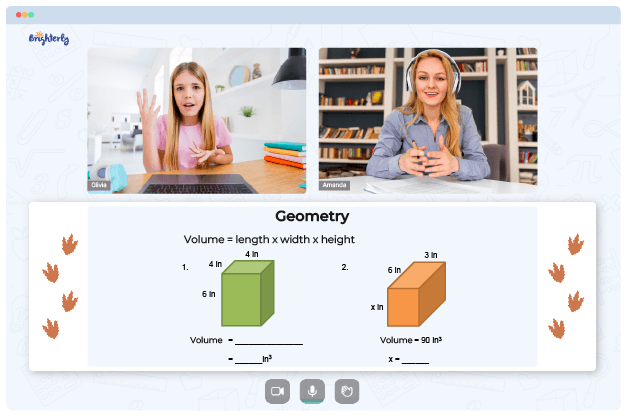# Classifying Triangles Worksheets

We’ll admit it: triangles can be pretty scary, not the shape itself but the sheer amount of triangles existing out there. We have different types of triangles, ranging from equilateral triangles to right-angled and obtuse-angled ones. While you might be able to keep track of these different types of triangles, this isn’t always the case with young learners. For a kid, it may be a tad overwhelming to recognize and determine the category each triangle belongs to.

But not for much longer. With a classifying triangles worksheet, your kid can become a pro at this topic in no time at all.

## About a Classify Triangles Worksheet

So, what are the triangle classification worksheets all about? Well, it’s quite simple. They are worksheets designed to enable students to learn how to identify triangles based on their sides, angles, or even both.

As we know, triangles can fall under two different classifications. Based on their angles, they may be classified into a right triangle, obtuse-angled triangle, or an acute triangle. However, based on their sides, they can be classified into an equilateral triangle, isosceles triangle, or a scalene triangle.

Math for Kids

Is Your Child Struggling With Math?
1:1 Online Math TutoringThe classifying triangles by angles worksheet will teach a kid more about these classifications and the ways to identify or analyze them. Students will also learn that an equilateral triangle has all equal sides, while an isosceles triangle has only two equal sides, and the scalene triangle has no equal sides at all.

More importantly, these worksheets come with answers to all the exercises. Although this may seem like a cheat sheet, it’s not. The aim of the classifying triangles worksheet answers is to help students cross-check their work and automatically grade their performance. Thus, it can come in really handy during self-led sessions.

## Benefits of a Classifying Triangles Worksheet PDF### Classifying Triangles Worksheets PDF

Classify Triangles Worksheet### Classifying Triangles Worksheets PDF### Classifying Triangles Worksheets PDF

Triangle Classification Worksheets### Classifying Triangles Worksheets PDF

Classifying Triangles Worksheet PDF

Wondering why you should download this worksheet? It’s simple, really. The classifying triangles worksheets provide deep insight into the classifications of triangles. Kids will learn how to identify triangles and use the right formulas to work with them.

The worksheet will help develop numerical and illustrating skills. Over time, children will be able to work with triangles faster and more efficiently.

### More Worksheets

Need help with Geometry?• Does your child struggle with understanding the fundamentals of geometry?
• Try studying with an online tutor.

Is your child having trouble mastering geometry? An online tutor could provide the necessary guidance.